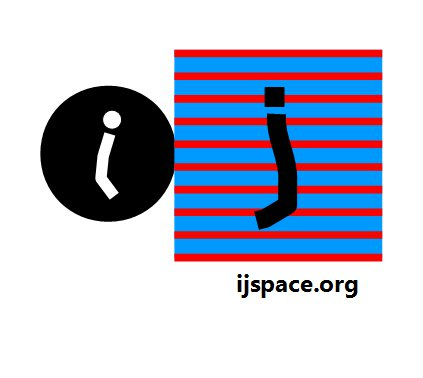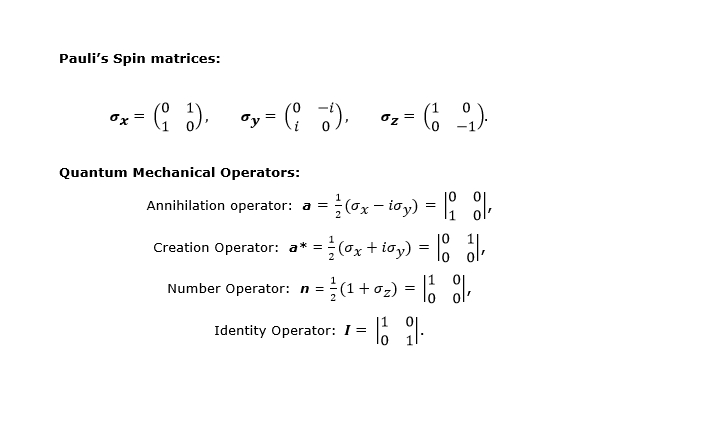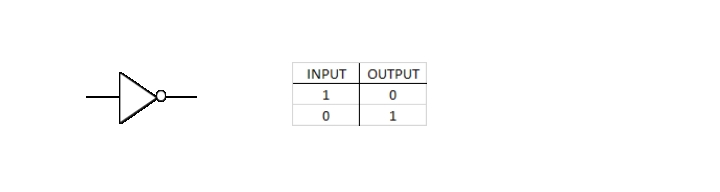# Home

 Quantum Computing- I What really is needed? 10th November 2017 "The rule of simulation that I would like to have is that the number of computer elements required to simulate a large physical system is only to be proportional to the space-time volume of the physical system. I don't want to have an explosion. That is, if you say I want to explain this much physics, I can do it exactly and I need a certain-sized computer. If doubling the volume of space and time means I'll need an exponentially larger computer, I consider that against the rules (I make up the rules, I'm allowed to do that)." - Richard P. Feynman   "A Quantum Computer is one whose operation exploits certain very specific transformations of its internal state.  The laws of quantum mechanics allow these peculiar transformations to take place under very carefully controlled (environmental and material) conditions." - N. David Mermin The "Quantum Computing" is being hailed as the next "disruptive technology", however very few people seem to have a clear understanding of why we need quantum computing and even if it becomes a reality in the near future, what will we do with it.  Usually the "Quantum Computing" is blended with all sorts of "Quantum Technologies" with expectations which are both unrealistic and vague at best.  So it is important that we separated out the quantum technologies and the quantum computing.  In this article our objective is to understand the requirements for the quantum computing.  Why do we need it?     The problem of simulating physical systems in nature, can be described as the problem of the “exact simulation”.  The conventional methodology is known as “approximate simulation” which represents the numerical simulation of differential equations.  The objective of quantum computing, is to evaluate the validity of the laws of physics with complete accuracy without any approximation.  These simulations obviously would be performed for the space-time volume.  But then how do we simulate time in digital computing?  In the same context, an important issue is the interpretation of the probability in complex space, in digital computing.     Feynman  described a two-state computational system based on the following operators which can be called "Quantum Gates":A system based on these operators is capable of simulating a discrete quantum system with limited numbers of degrees of freedom.  Theoretically such computational system, which is essentially based on the spin lattice, could provide required “exact” solutions for the physical systems observed in nature.  The problem to be solved, would use a computing architecture based on operators or quantum gates described above.  We do not know yet how this computing hardware would be designed.     However it is beneficial to understand what does the "exact simulation" or an exact solution means in terms of conventional silicon based digital computational architecture.  An exact solution means that given the output of a digital gate, the input to the gate can be precisely determined.  An example is an inverter or a NOT gate:In this case given the output the input can be accurately predicted.  This process is also known as "reversible computing".  Therefore for an exact simulation, we would need an universal digital computer capable of performing the reversible computing.  As it happens, an universal digital computer can be formed only by either NAND or NOR gates.  However neither NAND nor NOR gate, is capable of reversible computing as multiple input combinations are possible, for the same output.  There are various schemes suggested to overcome these limitations, but they add enormous complexities to the system and eventually scalability of the system hits its limit, a limit caused by finite resources available to us in (t, x, y, z) metric.  Therefore we have to think beyond the existing silicon based digital technology, if we want a true quantum computer. To be continued..Previous Blogs:   Insincere Symmetry - II Insincere Symmetry - I 3-D Infinite Source Nutshell-2016 Quanta-II Quanta-I EPR Paradox-II   EPR Paradox-I    Nutshell-2015